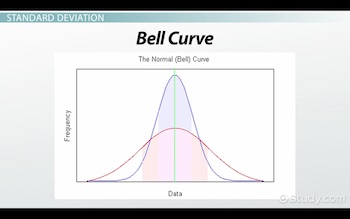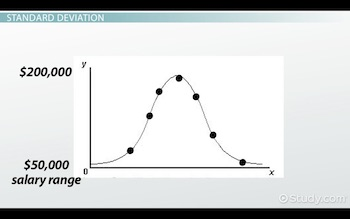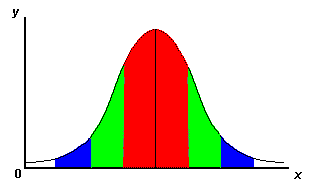# Standard Deviation in Psychology: Formula & Definition

An error occurred trying to load this video.

Try refreshing the page, or contact customer support.

Coming up next: Statistical Significance: Definition & Levels

### You're on a roll. Keep up the good work!

Replay
Your next lesson will play in 10 seconds
• 0:01 Standard Deviation
• 2:45 Deviations
• 3:58 Lesson Summary
Save Save

Want to watch this again later?

Timeline
Autoplay
Autoplay
Speed Speed

#### Recommended Lessons and Courses for You

Lesson Transcript
Instructor: Chevette Alston

Dr. Alston has taught intro psychology, child psychology, and developmental psychology at 2-year and 4-year schools.

Standard deviations are scores around the mean of a distribution. It measures how much a set of scores is dispersed around an average measure of variability. Deviations around the mean can be calculated to express it as a variance or a standard deviation.

## Standard Deviation

A standard deviation is a statistic that is calculated as the square root of a variance, or a data set calculated by taking the mean of the squared differences between each value and the mean value. Because the differences are squared, units of variance are not units of data. This is why a standard deviation is the square root of the variance. The points or units plotted from the variance becomes the data set. Standard deviations and variances are common measures of dispersion.

 σ = standard deviation σ ² = variance

## Average/Norm

A standard deviation is how a set of data is plotted around the mean (average) of a set of data. It is how a data set compares to a calculated norm or standard. The further the data spreads from the mean, the greater the deviation from the norm. Likewise, the closer the data are to the mean, the closer the data are to the norm, which creates a steep curve. The standard deviation of a data set that equals zero indicates all values in the set are the same.

## Bell Curve

Standard deviation data sets are plotted and dispersed around a bell curve. A bell curve is a symmetrical curve that represents the distribution, values, and frequencies in a set of data. From the middle point at the top, it slopes in a downward arc on both sides. The top middle point is the mean value or the maximum probability. Probability is likelihood of occurrence.## Distribution

As the probability decreases, the slope of the bell curve falls away from the mean. This explains why a wide deviation indicates a greater deviation from the mean. A normal distribution of data means that the numbers in the standard deviation's data set are close to the mean.

Units are plotted on the x-axis, or the horizontal line, in relation to how this information aligns with the frequency for each value on the y-axis, or the vertical line. For example, on the y-axis, salary, range may be from \$50,000 to \$200,000, with an average salary of \$200,000 (top of the bell curve). Let's say we plot the salaries of 30 people, or units, on the x-axis. The x-axis would reflect where each worker's salary would be plotted according to how much each person made. To the right of the median average, the worker would make more than \$200,000, and to the left of the median average, he or she would make less than \$200,000.## Deviations

One standard deviation from the mean (red area) on the x-axis usually accounts for about 68% of the data set. Two standard deviations from the mean (green area) usually accounts for about 95% of the data set. Likewise, three standard deviations from the mean (blue area) usually accounts for about 99% of the data set. Lastly, four deviations from the mean would be the white area.To unlock this lesson you must be a Study.com Member.

### Register to view this lesson

Are you a student or a teacher?

#### See for yourself why 30 million people use Study.com

##### Become a Study.com member and start learning now.
Back
What teachers are saying about Study.com

### Earning College Credit

Did you know… We have over 200 college courses that prepare you to earn credit by exam that is accepted by over 1,500 colleges and universities. You can test out of the first two years of college and save thousands off your degree. Anyone can earn credit-by-exam regardless of age or education level.